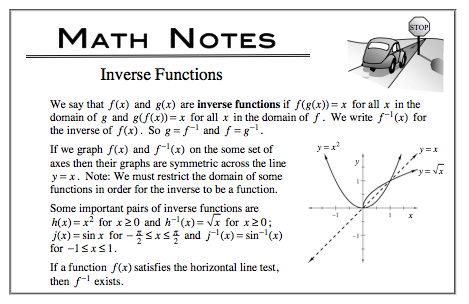### Home > CALC > Chapter 2 > Lesson 2.2.1 > Problem2-54

2-54.
1. If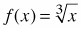, find: Homework Help ✎

1. f −1 (x)

2. f(f −1 (x))

3. f −1 (f(x))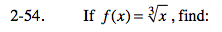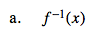$f(x)=\sqrt{x}$

$y=\sqrt{x}$

Now switch the x and y, and solve for y.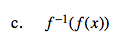Regarding parts (b) and (c), read the first sentence of the Math Note.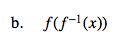$f(\text{part a})=\sqrt{\text{part a}}=$